# Finding Complex Zeros of a Polynomial Function

An error occurred trying to load this video.

Try refreshing the page, or contact customer support.

Coming up next: Finding Rational Zeros Using the Rational Zeros Theorem & Synthetic Division

### You're on a roll. Keep up the good work!

Replay
Your next lesson will play in 10 seconds
• 0:04 Polynomials
• 1:06 Zeros of Polynomials
• 2:08 Real Zeros on the…
• 2:47 Complex Zeros
• 4:45 Lesson Summary

Want to watch this again later?

Timeline
Autoplay
Autoplay
Speed

#### Recommended Lessons and Courses for You

Lesson Transcript
Instructor: Melanie Olczak

Melanie has taught high school Mathematics courses for the past ten years and has a master's degree in Mathematics Education.

This lesson will explain how to find complex zeros of a polynomial. You will be shown examples to apply factoring and the quadratic formula or square roots to solve for complex zeros.

## Polynomials

Have you ever been on a roller coaster? Did you know that the path of a roller coaster can be modeled by a mathematical equation called a polynomial? The up and down motion of a roller coaster can be modeled on the coordinate plane by graphing a polynomial. Imagine that you want to find the points in which the roller coaster touches the ground. These points are called the zeros of the polynomial. The zeros of a polynomial are also called solutions or roots of the equation.

A polynomial is a function that has multiple terms. Each term is made up of variables, exponents, and coefficients. Variables are letters that represent numbers. For polynomial functions, we'll use x as the variable. Coefficients are numbers that are multiplied by the variables. The degree of the polynomial is the highest exponent of the variable.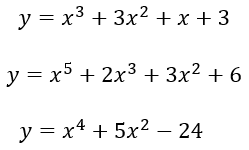## Zeros of Polynomials

As we mentioned a moment ago, the solutions or zeros of a polynomial are the values of x when the y-value equals zero. Polynomials can have real zeros or complex zeros. Real zeros to a polynomial are points where the graph crosses the x-axis when y = 0. When we graph each function, we can see these points. Complex zeros are the solutions of the equation that are not visible on the graph. Complex solutions contain imaginary numbers. An imaginary number is a number i that equals the square root of negative one. So complex solutions arise when we try to take the square root of a negative number.

The Fundamental Theorem of Algebra states that the degree of the polynomial is equal to the number of zeros the polynomial contains. So if the largest exponent is four, then there will be four solutions to the polynomial. If the largest exponent is a three, then there will be three solutions to the polynomial, and so on. Thinking in terms of the roller coaster, if it reaches the ground five times, the polynomial degree is five.

## Real Zeros on the Coordinate Plane

We can graph polynomial equations using a graphing calculator to produce a graph like the one below.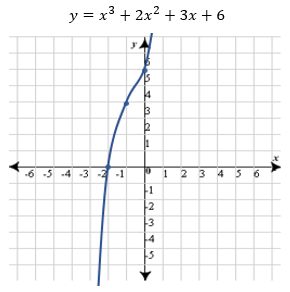Looking at this graph, we can see where the function crosses the x-axis. This graph has an x-intercept of -2, which means that -2 is a real solution to the equation.

Looking at the equation, we see that the largest exponent is three. This means the polynomial has three solutions. When we look at the graph, we only see one solution. How do we find the other two solutions?

Since the graph only intersects the x-axis at one point, there must be two complex zeros. In order to find the complex solutions, we must use the equation and factor.

## Complex Zeros

We will find the complex solutions of the previous problem by factoring. Since this polynomial has four terms, we will use factor by grouping, which groups the terms in a way to write the polynomial as a product of its factors.

1. First, we replace the y with a zero since we want to find x when y = 0. Then we group the first two terms and the last two terms.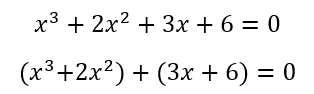2. Next, we look at the first two terms and find the greatest common factor. What numbers or variables can we take out of both terms? In the first set of parentheses, we can remove two x's. In the second set of parentheses, we can remove a 3.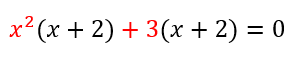3. Now, we group our two GCFs (greatest common factors) and we write (x + 2) only once.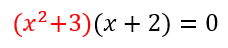To unlock this lesson you must be a Study.com Member.

### Register to view this lesson

Are you a student or a teacher?

### Unlock Your Education

#### See for yourself why 30 million people use Study.com

##### Become a Study.com member and start learning now.
Back
What teachers are saying about Study.com

### Earning College Credit

Did you know… We have over 160 college courses that prepare you to earn credit by exam that is accepted by over 1,500 colleges and universities. You can test out of the first two years of college and save thousands off your degree. Anyone can earn credit-by-exam regardless of age or education level.